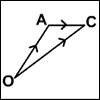#### You may also like### A Knight's Journey

This article looks at knight's moves on a chess board and introduces you to the idea of vectors and vector addition.### 8 Methods for Three by One

This problem in geometry has been solved in no less than EIGHT ways by a pair of students. How would you solve it? How many of their solutions can you follow? How are they the same or different? Which do you like best?### An Introduction to Vectors

The article provides a summary of the elementary ideas about vectors usually met in school mathematics, describes what vectors are and how to add, subtract and multiply them by scalars and indicates why they are useful.

# From Point to Point

##### Age 14 to 16 ShortChallenge Level

How can you get from $(10,66)$ to  $(20,12)$

using only integer multiples of the vectors

$\begin{pmatrix}2\\-3\end{pmatrix}$ and $\begin{pmatrix}3\\2\end{pmatrix}$?

If you liked this problem, here is an NRICH task which challenges you to use similar mathematical ideas.
You can find more short problems, arranged by curriculum topic, in our short problems collection.